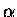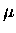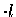Next: Discussion of stopping fraction Up: Absolute amplitude method Previous: Delayed electron lifetime

## Delayed electron cuts efficiency

The efficiencies for the delayed electron and telescope cuts were determined from the ratio of thesignal yields with and without the Del cuts. From each spectrum the background was subtracted, usually using a pure H2 target in which no fusion takes place. Theevents were selected with an energy cut of [2000, 3700] ch, and a time cut of [0.02, 0.4]s was applied to the Si signals.

Columns 3 to 5 in Table 6.17 show the Del cut efficiency,for Si1, Si2, and their average. Run A is the same as that in Tables 6.14 and 6.15. Run C was similar to A but had no downstream layers (500 TH2 and 3 TD2 were present for Run A). Run D had a 6 Tupstream overlayer (as opposed to 14 Tas in others), testing possible effects of the layer thickness and the reaction depth. Given in brackets forare statistical errors. Run A had sufficient statistics, while Runs C and D, as well as Del-t cuts (Tel1, Tel2) had relatively poor statistical precision. Some variations of the Del cut efficiencies are observed in Table 6.17: Run C and D have a lowerthan A by 2 to 3, possibly pointing to a systematic effect due to the target conditions.

The interpretation of theresults requires some caution; recall that in the present analysis, detection of the fusion reactions is used to tag the muon as a well-defined source for the electron, into which it decays. Detecting the fusion, however, biases the electron source to preferentially concentrate towards the region where the acceptance for the particular Si detector is higher, e.g., the beam-left edge of the target for Si1 (see Fig. 3.18, page). The overall efficiency of the Del cuts, which is a convolution of theparticle acceptance in the Si detector and the electron acceptance in the scintillators (telescope), is thus higher in the case of the Del coincidence in the same side of the target than that in the opposite side (Fig 3.18); e.g., for Si1,is higher for Ege at the beam left than En1 at the beam right, as can be seen in Table 6.17. This acceptance bias effect can be largely avoided by taking the average of Si1 and Si2, yet the effect persists to increase(unless the target is infinitely small), and is at the several percent level in our case.

On the other hand, the downstream electron detectors (En2, Tel2), which were on the beam (Z) axis (as opposed to the perpendicular axis (X)), are less susceptible to the bias effect, since they have relatively uniform acceptance over the entire target. This can be seen in the consistency between Si1 and Si2 infor En2 and Tel2 in Table 6.17. En2 and Tel2, which have lower values of, are thus more reliable compared to other detectors.

With all the factors in Eq. 6.26 determined, the stopping fraction with the absolute yield method can now be computed and the results are given in the last column of Table 6.17. The error includes 3.6% systematic uncertainty due to the delayed electron life time, , which dominates the total error for Run A, but is comparable to the statistical error for Runs C and D. The values in bold face are for the detectors downstream (En2, Tel2), which are more reliable than other detectors, as discussed above. Among these SFABSHvalues for En2 and Tel2, there are about % variations depending on the target conditions or the choice of the detector (i.e., En2 versus Tel2), which indicates a measure of further systematic effects. Note that unlike the case for the relative amplitude method, in which there was some 30% discrepancy in between the scintillators and telescopes (see Table 6.6 in pageand 6.8 in page), the results in the two detectors are nearly consistent in the absolute yield method here. Taking the average of the two extremes of the downstream SFABSH, we quote , where with the assumption that the uncertainty is dominated by the systematics, the 5% error is given by a quadratic addition of the 3.6% systematic error from each measurement and 3.3% due to run variations.Next: Discussion of stopping fraction Up: Absolute amplitude method Previous: Delayed electron lifetime